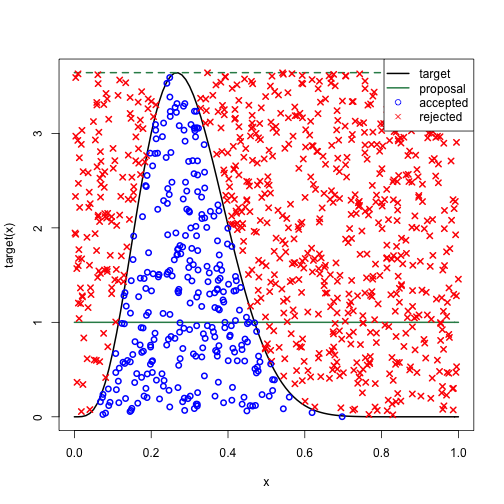Here is a quick example of rejection sampling with the example coming almost verbatim from Robert and Casella. Suppose f(x) is our target distribution and q(x) is our proposal distribution. Given an M such that f(x) <= M q(x) for all x, rejection sampling samples a value x from q(x) and U~Unif(0,1) and accepts it if U <= f(x)/M q(x). The probability of acceptance is 1/M.

In this example, we have a Beta(a,b) as our target distribution and the proposal distribution is Unif(0.1). The M that attains the above inequality is simply the density of the Beta(a,b) at its mode. Since the mode is c=(a-1)/(a+b-2), M = Beta(c;a,b).

Set up the targt and proposal densities.

Calculate M and the probability of acceptance.

Perform rejection sampling

The plot below has target (red) and proposal (green) density as well as the proposal density scaled by M (green, dashed) to show how it creates an envelope over the target. The points are accepted (blue circle) and rejected (red x) values on the x-axis with their associated uniform draws on the y-axis.The empirical acceptance probability is.